Courses

# Chapter - 1 Physical Chemistry Class 12 Notes | EduRev

## Class 12 : Chapter - 1 Physical Chemistry Class 12 Notes | EduRev

The document Chapter - 1 Physical Chemistry Class 12 Notes | EduRev is a part of the Class 12 Course Chemistry Class 12.
All you need of Class 12 at this link: Class 12

ATOMIC STRUCTURE

Estimation of closest distance of approach (derivation) of α-particle :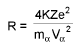The radius of a nucleus : R = R0 (A)1/3 cm

Planck's Quantum Theory : Energy of one photon = hv = (hc/λ)

Photoelectric Effect: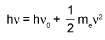Bohr’s Model for Hydrogen like atoms :

1. mvr = n(h/2π) (Quantization of angular momentum)
2.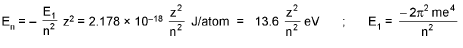3.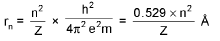4.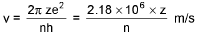De-Broglie wavelength :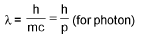Wavelength of emitted photon :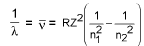No. of photons emitted by a sample of H atom :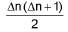Heisenberg’s uncertainty principle: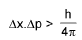Or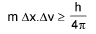Or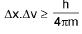Quantum Numbers:

• Principal quantum number (n) = 1,2, 3, 4 .... to∞.
• Orbital angular momentum of electron in any orbit = nh/2π.
• Azimuthal quantum number (l) = 0 , 1 .... to (n - 1).
• Number of orbitals in a subshell = 2l + 1
• Maximum number of electrons in particular subshell = 2x (2l+ 1)
• Orbital angular momentum L =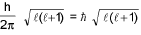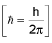STOICHIOMETRY

• Relative atomic mass (R.A.M)=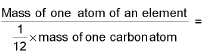Total Number of nucleons
• Y-map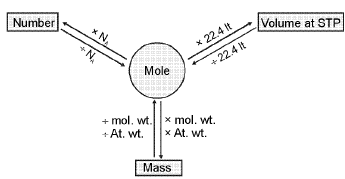Density: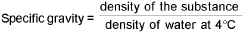For gases:

Absolute density (mass/volume) =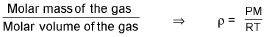Vapour density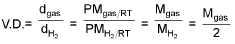Mgas = 2 V.D.

Mole-mole analysis: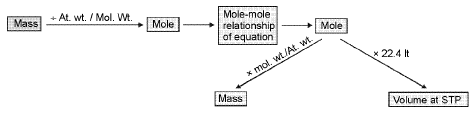Concentration terms:

Molarity (M):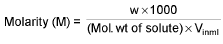Molality (m):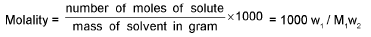Mole fraction (x):

∴ Mole fraction of solution (x1) =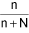∴ Mole fraction of solvent (x2) =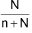% Calculation: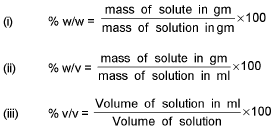Derive the following conversion :

1. Mole fraction of solute into molarity of solution M =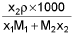2. Molarity into mole fraction x2 =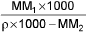3. Mole fraction into molality m =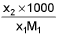4. Molality into mole fraction x2 =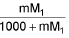5. Molality into molarity M =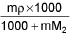6. Molarity into Molality m =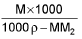M1 and M2 are molar masses of solvent and solute, ρ is density of solution (gm/mL)

M = Molarity (mole/lit.), m = Molality (mole/kg), x1 = Mole fraction of solvent, x2 = Mole fraction of solute

Average/Mean atomic mass :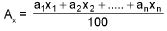Mean molar mass or molecular mass: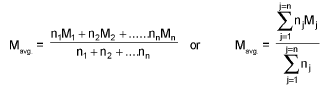Calculation of individual oxidation number :

Formula: Oxidation Number = number of electrons in the valence shell - number of electrons left after bonding

Concept of Equivalent weight/Mass :

For elements, equivalent weight (E) =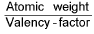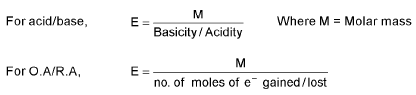Equivalent weight (E) =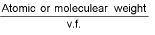(v.f. = valency factor)

Concept of number of equivalents :

No. of equivalents of solute =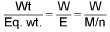No. of equivalents of solute = No. of moles of solute x v.f.

Normality (N):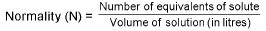Normality = Molarity x v.f.

Calculation of valency Factor :

n-factor of acid = basicity = no. of H+ ion(s) furnished per molecule of the acid,

n-factor of base = acidity = no. of OH- ion(s) furnised by the base per molecule.

At equivalence point: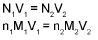Volume strength of H2O2:

20V H2O2 means one litre of this sample of H2O2 on decomposition gives 20 It. of O2 gas at S.T.P.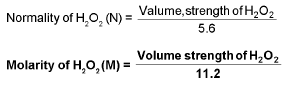Measurement of Hardness: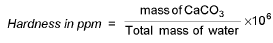Calculation of available chlorine from a sample of bleaching powder :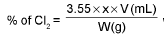where x = molarity of hypo solution and v = mL. of hypo solution used in titration.

Offer running on EduRev: Apply code STAYHOME200 to get INR 200 off on our premium plan EduRev Infinity!

## Chemistry Class 12

121 videos|250 docs|199 tests

,

,

,

,

,

,

,

,

,

,

,

,

,

,

,

,

,

,

,

,

,

;